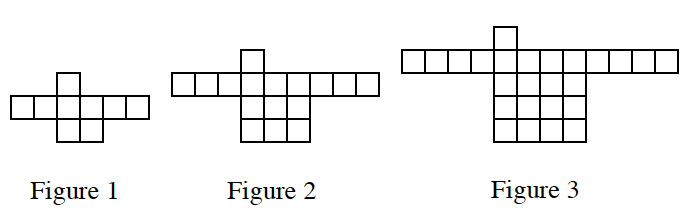### Home > CC3 > Chapter 4 > Lesson 4.1.1 > Problem4-2

4-2.

For each tile pattern in problem 4-1, draw Figures  $0$, $4$, and $5$ on graph paper. If it helps, copy Figures $1$, $2$, and $3$ onto your paper. Homework Help ✎

1.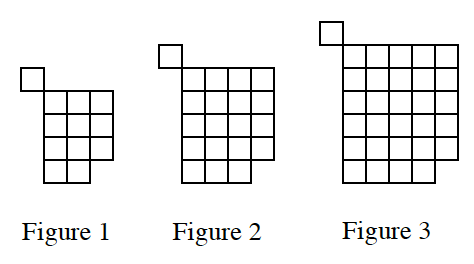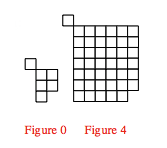1.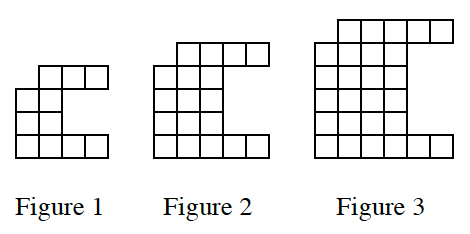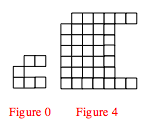1.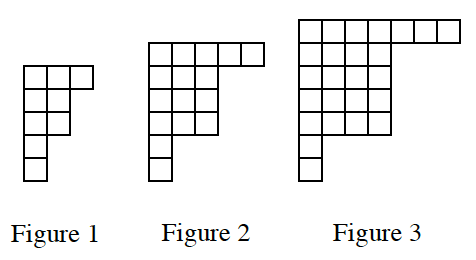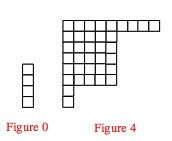1.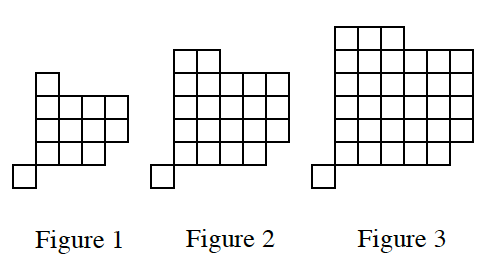Try parts (d) and (e) on your own following the method used above.
Remember to draw Figure $5$ for the partial answers given above.

1.